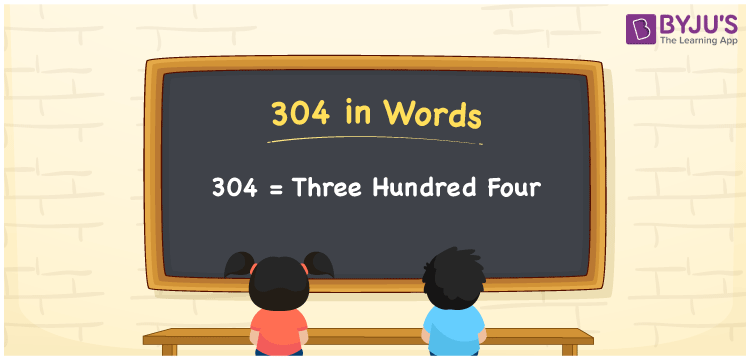# 304 in Words

304 in words can be written as Three Hundred Four. Suppose if you purchase one kg of sugar for Rs. 304, then you can say that “I purchased 1 kg of sugar for Three Hundred Four Rupees”. The place value of the three digits can be understood with the help of the table provided for reference here. Using it, students will be able to write the numbers in words with ease. Hence, 304 can be read as “Three Hundred Four” in words.

 304 in words Three Hundred Four Three Hundred Four in Numbers 304

## 304 in English Words## How to Write 304 in Words?

Let us prepare the place value chart having three columns indicating the digits in 304. Using the chart, writing numbers in words will be very easy.

 Hundreds Tens Ones 3 0 4

The expanded form of 304 is provided below:

3 x Hundred + 0 × Ten + 4 × One

= 3 x 100 + 0 x 10 + 4 x 1

= 300 + 4

= 304

= Three Hundred Four

Therefore, 304 in words is written as Three Hundred Four.

304 is a natural number that precedes 305 and succeeds 303.

304 in words – Three Hundred Four

Is 304 an odd number? – No

Is 304 an even number? – Yes

Is 304 a perfect square number? – No

Is 304 a perfect cube number? – No

Is 304 a prime number? – No

Is 304 a composite number? – Yes

## Frequently Asked Questions on 304 in Words

Q1

### How do you write the number 304 in words?

304 in words is Three Hundred Four.
Q2

### Find the value of 300 plus 4.

The value of 300 plus 4 is 304. Therefore, 304 in words is Three Hundred Four.
Q3

### Is 304 a perfect square?

No, 304 is not a perfect square as it cannot be expressed as the product of two integers which are equal.